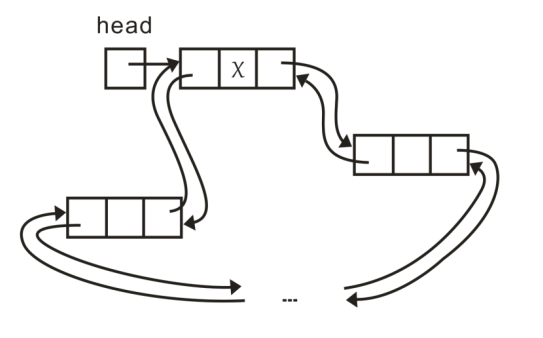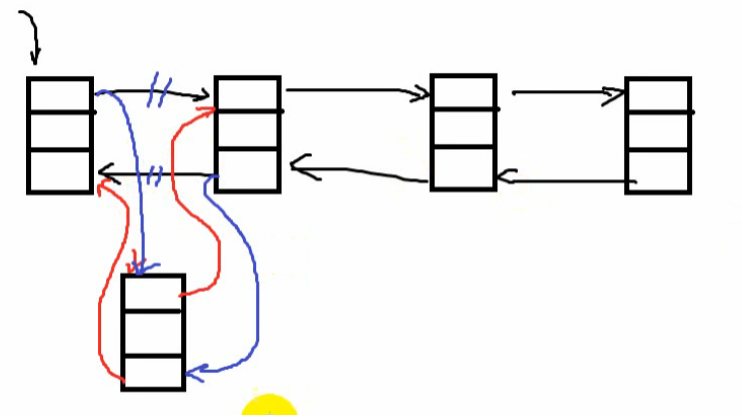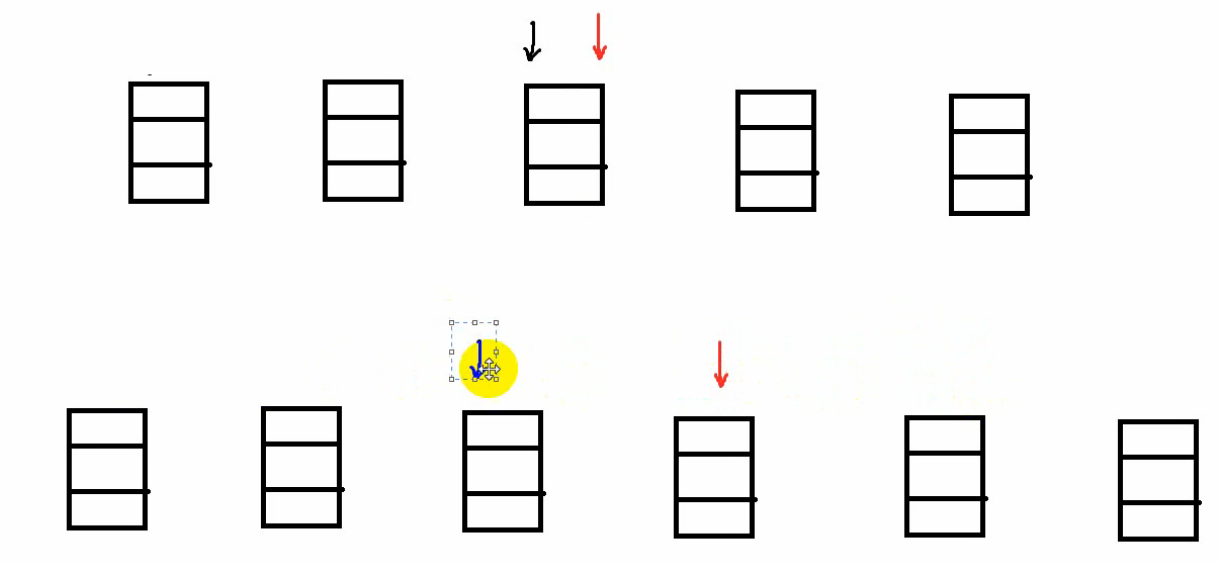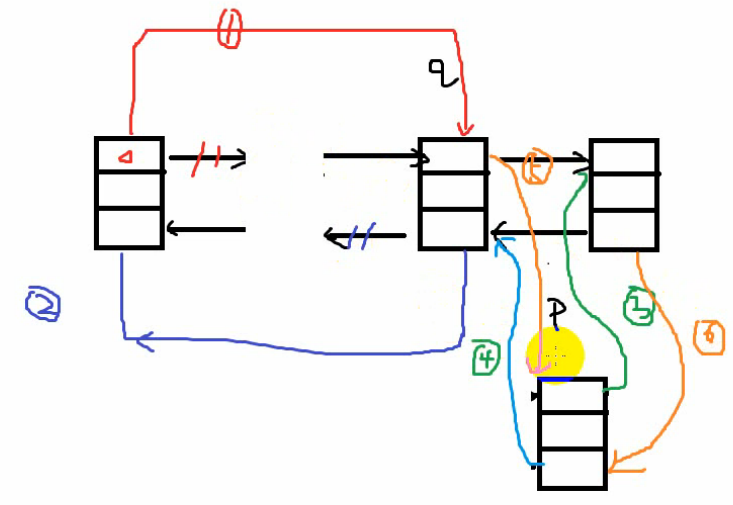# c++ 双向链表#include <iostream>
using std::cout;
using std::endl;
struct Node
{
int data;
struct Node * next;
struct Node * pre;
};


Node * createList()
{
Node * head = new Node;
if (NULL == head)
exit(-1);
head->next = head;
head->pre = head;
return head;
}void insertList(Node * head,int n)
{
Node * cur = new Node;
if (NULL == cur)
exit(-1);
cur->next = head->next;
cur->pre = head;
head->next = cur;
cur->next->pre = cur;

cur->data = n;
}


int lenList(Node * head)
{
int i = 0;
Node * t = head->next;
while (t != head)
{
i++;
t = t->next;
}
return i;
}Node * findList(Node * head,int fn)
{
Node * forward = head->next;
Node * back = head->pre;
while (forward != back->next)
{
if (forward->data == fn)
return forward;
if (back->data == fn)
return back;
if (forward == back)
break;
forward = forward->next;
back = back->pre;
}
return NULL;
}


void deleteList(Node * pFind)
{
pFind->pre->next = pFind->next;
pFind->next->pre = pFind->pre;
delete pFind;
}


（类似于先删除 再插入）void sortDlist(Node * head)
{
int len = lenList(head);
Node *prep = NULL;
Node *p = NULL;
Node *q = NULL;
Node *t = NULL;
for (int i = 0;i < len - 1;i++)
{
p = head->next;
q = p->next;
for (int j = 0;j < len - 1 - i;j++)
{
if ((p->data)<(q->data))
{
p->pre->next = q;
q->pre = p->pre;

p->next = q->next;
p->pre = q;

q->next = p;
p->next->pre = p;

t = p;
p = q;
q = t;
}
p = p->next;
q = q->next;
}
}
}


void desList(Node * head)
{
head->pre->next = NULL;
Node *t = NULL;
while (head != NULL)
{
t = head;
head = head->next;
delete t;
}
}06-09
03-07
08-19
08-23
11-01
04-22
11-26
03-31
12-02
05-25
04-095981
09-28
©️2020 CSDN 皮肤主题: 大白 设计师:CSDN官方博客